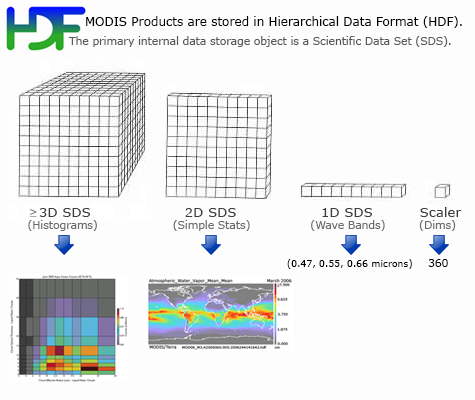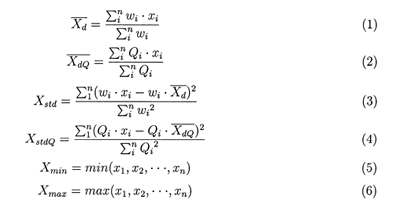Sorry, you need to enable JavaScript to visit this website.# Format & Content

Monthly Product (08_M3) File Format BasicsMODIS Atmosphere Monthly (08_M3) product files are stored in Hierarchical Data Format (HDF). HDF is a multi-object file format for sharing scientific data in multi-platform distributed environments. HDF files should only be accessed through HDF library subroutine and function calls, which can be downloaded from the HDF web site.

Each of the global gridded statistical fields within the HDF file is stored as a Scientific Data Set (SDS). L3 Atmosphere products contain hundreds of 1°×1° global gridded Scientific Data Sets (SDSs) or statistics derived from up to 31 consecutive days of the Daily (08_D3) product.  (The Daily product is derived from L2 products of Aerosol (04_L2), Water Vapor (05_L2), Cloud (06_L2), and Atmospheric Profile (07_L2)). The grid domain or size is 360x180 pixels, which corresponds to 360 degrees of longitude and 180 degrees of latitude.

There are a number of general L3 Monthly algorithm characteristics:

• Only the L3 Daily files are used as input. (Greatly improves algorithm efficiency.)
• L3 Daily and L3 Multiday HDF files use an identical grid, SDS dimensions, and histogram bin definitions. (This is an algorithm requirement.)
• There is no “valid range” check, only “Fill_Value” L3 Daily (08_D3) grid cells are universally excluded from L3 Multiday statistics.
• One of three weighting schemes are used to compute Monthly statistics from Daily statistics:
1. Unweighted, where each L3 Daily grid cell is given equal weight in the L3 Multiday mean. This is a simple time-averaged mean, useful for computing such things as temperature averages over a multi-day period.
2. Pixel Count Weighted, where each L3 Daily grid cell is weighted by the valid L2 Pixel Counts in that grid. This is a count-averaged mean that ensures computed means match means computed from histograms -- useful for example, for cloud optical properties.
3. Min Num Days (Aerosol derived products only), where each L3 Daily grid cell is given equal weight in the L3 Multiday mean, however there is a requirement that at least three (3) days have a valid data value. This is a simple time-averaged mean, but requires at least 3 days to have valid data. This is a new weighting scheme implemented in Collection 061 (6.1), it's useful for computing Aerosol statistics over a monthly period, which minimized cloud and snow/ice contamination of the Monthly Aerosol statistics.

Monthly Product File (08_M3) Parameter List (primary parameters only)

Monthly Product (08_M3) Statistic List

A number of statistical summaries are computed, depending on the science parameter being considered. Statistics for a given Level-2 measurement might include:

Simple Statistics of the Mean

• Mean_Mean
• Mean_Min
• Mean_Max
• Mean_Std

Mean of the Standard Deviation

• Std_Deviation_Mean

QA Weighted Statistics

• QA_Mean_Mean
• QA_Mean_Std
• QA_Mean_Min
• QA_Mean_Max
• QA_Std_Deviation_Mean

Histograms of the quantity within each grid box

• Histogram_Counts

Histograms of the confidence placed in each measurement

• Confidence_Histograms

Mean and Standard Deviation of the Fraction of pixels that satisfy some condition (e.g. cloudy, clear)

• FMean
• FStd

Total number of observations (pixels) within each grid box

• Pixel_Counts

Parameters of Log-Normal Distributions

• Log_Mean_Mean
• Log_Mean_Std
• Log_Mean_Min
• Log_Mean_Max
• Log_Std_Deviation_Mean

Parameters of QA Weighted Log-Normal Distributions

• QA_Log_Mean_Mean
• QA_Log_Mean_Std
• QA_Log_Mean_Min
• QA_Log_Mean_Max
• QA_Log_Std_Deviation_Mean

Regressions and/or Histograms derived from comparing one science parameter to another, statistics may be computed for a subset that satisfies some condition

• Joint_Histogram_vs_Temperature
• Joint_Histogram_vs_Emissivity
• Joint_Histogram_47vs55
• Joint_Histogram_47vs66
• Joint_Histogram_55vs66

Monthly Product (08_M3) Statistic Computation Details

Below are more details on the computation of each statistic:

For the multiday product, assume that x1, x2, ..., xn represent the L3 Daily value of a parameter over a 1 x 1 degree grid box, wi is the weighting factor (1 for the unweighted case and the pixel count value for the pc-weighted case), then the simple statistics are defined as:In these equations, (1) will be referred to as the 'regular' mean, (2) not computed in the multiday, (3) as the regular standard deviation, (4) not computed in the multiday, (5) as the minimum, and (6) as the maximum.

The simple statistics also include daily log regular mean, log standard deviation, log QA mean, and log QA standard deviation. These log quantities are calculated as shown in equations (1) and (3), except that x1, x2, ..., xn are replaced by their logarithms.

The histograms and joint histograms report the counts of the pixels falling into predetermined numerical intervals.

The pixel counts are used to represent the number of pixels for the parameters which do not have QA flags, while the confidence-histograms-counts are used to represent the number of counts for each parameter that falls within each QA bin (e.g., questionable, good, very good, and total).